# Logic

A man can drink a barrel of water for 26 days, woman for 48 days. How many days will a barrel last between them?

Correct result:

x =  16.865 d

#### Solution:

x•(1/26 + 1/48) = 1

37x = 624

x = 62437 ≈ 16.864865

Calculated by our simple equation calculator.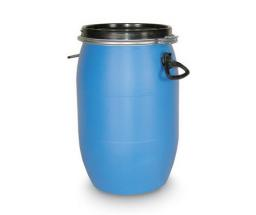Our examples were largely sent or created by pupils and students themselves. Therefore, we would be pleased if you could send us any errors you found, spelling mistakes, or rephasing the example. Thank you!

Please write to us with your comment on the math problem or ask something. Thank you for helping each other - students, teachers, parents, and problem authors.Math student
Very poor English! Who phrased this problem(?) as it is not stated correctly - it is incoherent!

1 year ago  2 LikesMath student
Extremely poor wording! A man drinks a barrel of water for 26 days, woman for 48 days, for how many days they drink barrel together? Who wrote this???? It should be, a man can drink...., and a woman...., how many days will a barrel last between them (or for both of them). Who ever wrote this question has very poor English skills and should not be writing math word problems using English!

1 year ago  1 LikeTips to related online calculators
Looking for calculator of harmonic mean?
Looking for a statistical calculator?
Need help calculate sum, simplify or multiply fractions? Try our fraction calculator.
Do you have a linear equation or system of equations and looking for its solution? Or do you have quadratic equation?
Do you want to convert time units like minutes to seconds?

## Next similar math problems:

• Sow barley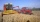Farmers wanted to sow barley within 13 days. Due to the excellent weather, they managed to exceed the daily plan of sowing by 2 ha and therefore finished sow grain in 12 days. How many hectares of land did they sow with barley?
• Wooden blockA cuboid-shaped wooden block has 6 cm length, 4 cm breadth, and 1 cm height. Two faces measuring 4 cm x 1 cm are colored in black. Two faces measuring 6 cm x 1 cm are colored in red. Two faces measuring 6 cm x 4 cm are colored in green. The block is divid
• Gas sphere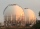The gas tank has the shape of a sphere with a diameter of 14 m. How many m3 of gas will fit in it?
• Magic bagEach time the prince crossed the bridge, the number of tolars in the magic bag doubled. But then the devil always conjured 300 tolars for him. When this happened for the third time, the prince had twice as much as he had in the beginning. How many tolars
• Big numbersHow many natural numbers less than 10 to the sixth can be written in numbers: a) 9.8.7 b) 9.8.0
• You have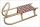You have 4 reindeer and you want to have 3 fly your sleigh. You always have your reindeer fly in a single-file line. How many different ways can you arrange your reindeer?
• Clay balls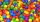How many clay balls with a radius of 1 cm can be made from a ball of clay with a radius of 8 cm?
• Chimney and treeCalculate the height of the factory chimney, which casts a shadow 6.5 m long in the afternoon. At the same time, a 6 m high tree standing near it casts a shadow 25 dm long.
• The regularThe regular triangular prism has a base in the shape of an isosceles triangle with a base of 86 mm and 6.4 cm arms, the height of the prism is 24 cm. Calculate its volume.
• Similar trianglesTriangle A'B'C 'is similar to triangle ABC, whose sides are 5 cm, 8 cm, and 7 cm long. What is the length of the sides of the triangle A'B'C ' if its circumference is 80 cm?
• Hexagonal pyramidFind the area of a shell of the regular hexagonal pyramid, if you know that its base edge is 5 cm long and the height of this pyramid is 10 cm.
• Company carMs. Vaňková has a company car equipped with a multifunction indicator, on which she can monitor the average petrol consumption during individual rides. During the first ride, mostly in city traffic, it traveled 20.5 km, with an average consumption of 7.8L
• Similar trianglesThe triangles ABC and XYZ are similar. Find the missing lengths of the sides of the triangles. a) a = 5 cm b = 8 cm x = 7.5 cm z = 9 cm b) a = 9 cm c = 12 cm y = 10 cm z = 8 cm c) b = 4 cm c = 8 cm x = 4.5 cm z = 6 cm
• ParallelogramFind the perimeter of the parallelogram, where base a = 8 cm, height v = 3 cm, and angle alpha = 35° is the magnitude of the angle at vertex A.
• Edge of prismThe regular quadrilateral prism has a surface of 250 dm2, its shell has a content of 200 dm2. Calculate its leading edge.
• Truncated cone 6Calculate the volume of the truncated cone whose bases consist of an inscribed circle and a circle circumscribed to the opposite sides of the cube with the edge length a=1.
• Value of expressionX=2, y=-5 and z=3 what is the value of x-2y?